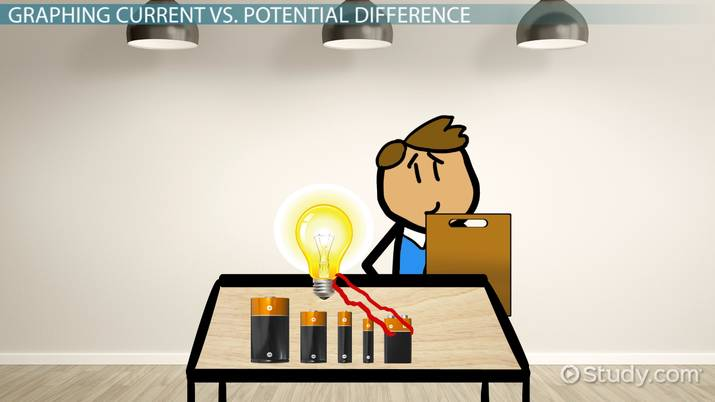Science Courses / Course / Chapter

# Ohm's Law and Voltage vs. Current Graphs

Christina Keathley, Betsy Chesnutt
• Author
Christina Keathley

Christina graduated with a Master's in biology from the University of Louisiana at Lafayette. She is a current PhD student in biology at Wake Forest University, and has been teaching undergraduate students biology for the last three years.

• Instructor
Betsy Chesnutt

Betsy has a Ph.D. in biomedical engineering from the University of Memphis, M.S. from the University of Virginia, and B.S. from Mississippi State University. She has over 10 years of experience developing STEM curriculum and teaching physics, engineering, and biology.

Understand the voltage vs. current graph. Learn about Ohm's law, Ohm's law graph, various examples, and the significance of the slope of voltage vs. current graphs. Updated: 06/17/2022

Show

## Voltage vs. Current Graph

There are multiple units of measurement that are used to describe an electrical system. For instance, current and voltage are two forms of measurement used to describe properties of electricity that are often confused with one another. Current can be described as the flow of charges. For instance, if electricity were a falling ball, current would be the speed at which the ball passes a determined point between its drop and land point. Voltage is often compared to force. Using the same analogy, voltage would be the gravity that is pulling the ball towards the ground. These differences are summarized in the following table:

Type of Liquid Voltage Current
Definition Force or pressure pushing electricity through a circuit Rate at which electricity passes through a point in a circuit
If a ball was dropped... Voltage is the gravity pulling the ball towards the ground Current is the speed at which a ball is falling at a given point
Units Volts (V) Amps (A)
Equation V=IR I=V/R

Voltage, current, and resistance are all related. Resistance (measured in Ohms) prevents current from being able to flow. If a ball were dropped into water, it would not reach the floor as quickly as if it were dropped in air because the water creates resistance. The relationship between resistance (R), current (I), and voltage (V) can be described mathematically:

{eq}V=IR {/eq}

In this equation, voltage is also referred to as the potential difference, which is the difference in energy occurring at two different points along a circuit. Voltage and current can be graphed on a voltage vs. current graph, which depicts voltage on the x-axis and current on the y-axis. A voltage vs. current graph may be portrayed as a current vs. potential difference graph. In this instance, the x-axis represents the current and the y-axis represents the current.

### Voltage, Current, and Resistance Example

Michael is calculating the resistance in his circuit. Using the proper equipment, he measures the amplitude and voltage of the system. Michael finds that his circuit has a voltage of 0.4V and a current of 0.7A. Knowing that the material he uses supports a linear relationship between voltage and current, he calculates the following resistance:

{eq}V=IR {/eq}

{eq}0.4=0.7*R {/eq}

{eq}\frac{0.4}{0.7}=R {/eq}

{eq}R=0.57 {/eq}

Using the provided equation, Michael finds that his circuit has a resistance of 0.57 Ohms.

### Slope of Voltage vs. Current Graph

The slope of a voltage vs. current graph provides important information about a circuit. When the slope of the line is equal to 1, then the current and voltage increase proportionally. In some instances, the line may be a horizontal or vertical line. If the line is horizontal, then the current remains the same regardless of the change in voltage. Instead, voltage and resistance change. If the line is vertical, then even when the voltage is not changing, resistance and current are.The slope of a graph is equal to the change in its rise over the change in its run. Because resistance is equal to the voltage divided by the current, the slope of a voltage vs. current graph is the inverse of the resistance. In a current vs. potential difference graph, the slope is equal to the resistance.An error occurred trying to load this video.

Try refreshing the page, or contact customer support.

Coming up next: Finding the Equivalent Resistance: Series, Parallel & Combination Circuits

### You're on a roll. Keep up the good work!

Replay
Your next lesson will play in 10 seconds
• 0:00 Graphing Current vs.…
• 1:00 Ohm's Law
• 2:05 Ohmic vs. Non-Ohmic Materials
• 2:42 Lesson Summary
Save Save

Want to watch this again later?

Timeline
Autoplay
Autoplay
Speed Speed

To unlock this lesson you must be a Study.com Member.

#### What does the slope of the current vs. voltage graph give?

The slope of the voltage vs. current graph is equivalent to the inverse of the resistance. The slope of the current vs. potential difference graph is equal to the resistance.

#### What is the relationship between voltage and current?

Current, voltage, and resistance are related through Ohm's Law. Voltage is equal to the resistance of a circuit multiplied by its current.

#### What is the relationship between current and voltage graphs?

Voltage and current may be depicted on voltage vs. current graphs, where voltage is on the x-axis and current is on the y-axis. Voltage may be reflected on the y-axis as potential difference in current vs. potential difference graphs.

### Register to view this lesson

Are you a student or a teacher?

Back

### Resources created by teachers for teachers

Over 30,000 video lessons & teaching resources‐all in one place.Video lessonsQuizzes & WorksheetsClassroom IntegrationLesson Plans

I would definitely recommend Study.com to my colleagues. It’s like a teacher waved a magic wand and did the work for me. I feel like it’s a lifeline.

Jennifer B.
TeacherCreate an account to start this course today
Used by over 30 million students worldwide